### 15.2.10. Panel and Flange Buckling Summary

Reference: Abbott, Richard. Analysis and Design of Composite and Metallic Flight Vehicle Structures 3 Edition, 2019.

15.2.10.1 Rectangular Panel Buckling Coefficients

(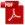NACA-TN-3781, 1957) gives the following summary for minimum buckling coefficient figures (infinitely long Panels):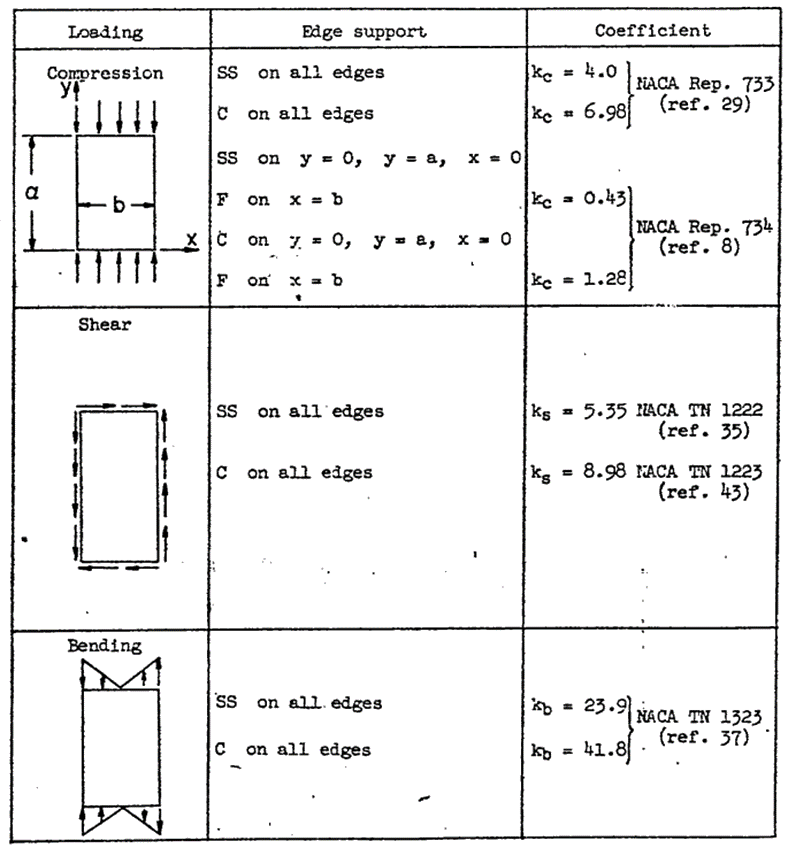Figure 15.2.10‑1: Rectangular Panel and Flange Buckling Stress Coefficient Summary  (NACA-TN-3781, 1957)

15.2.10.2. Rectangular Panel Plasticity Correction Factors

And the following summary for plasticity reduction factors: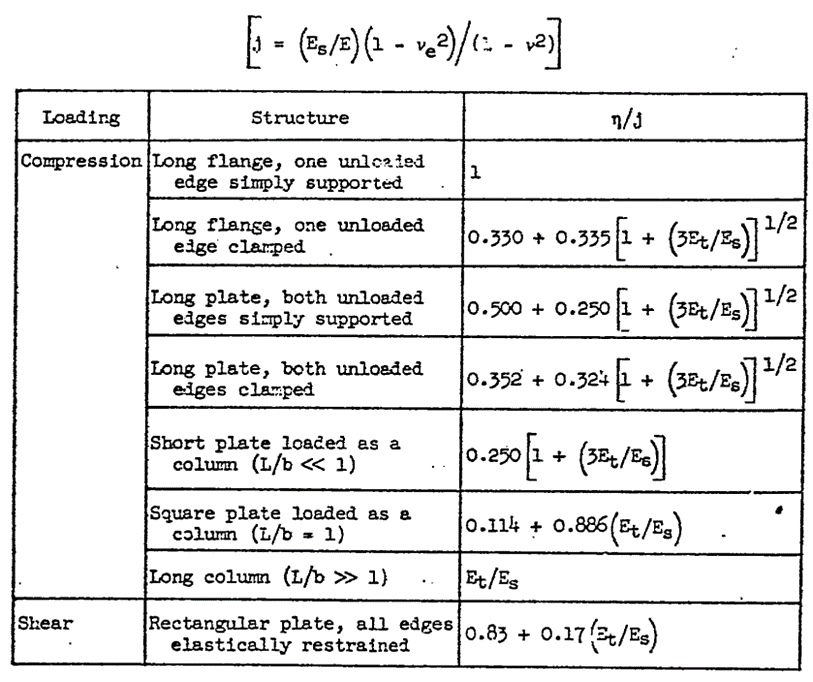Figure 15.2.10‑2: Rectangular Panel and Flange Buckling Plasticity Correction Factors  (NACA-TN-3781, 1957)

15.2.10.3. Rectangular Panel Cladding Correction Factors

And the following summary for cladding reduction factors: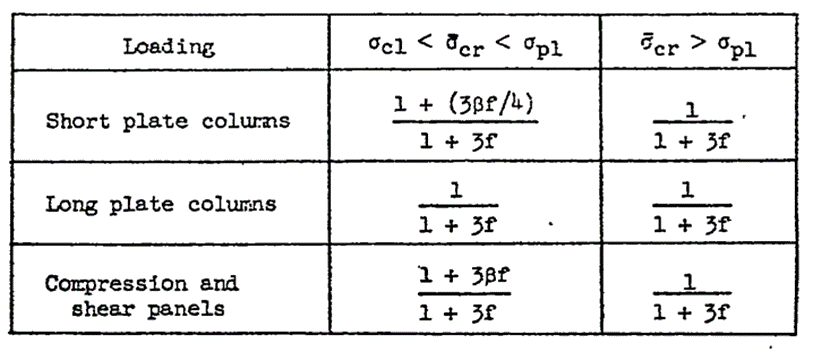Figure 15.2.10‑3: Rectangular Panel and Flange Buckling Cladding Correction Factors  (NACA-TN-3781, 1957)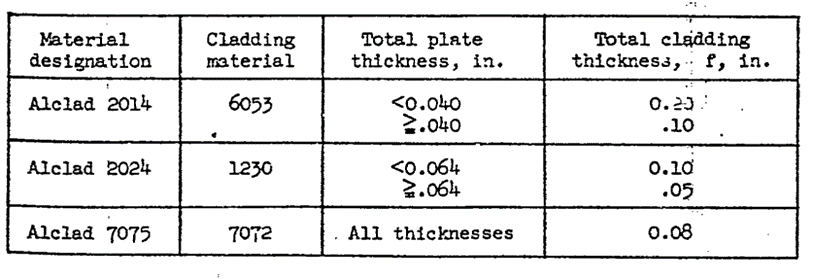Figure 15.2.10‑4: Rectangular Panel and Flange Buckling Cladding Thicknesses  (NACA-TN-3781, 1957)

15.2.10.4. Rectangular Panel Buckling Interactions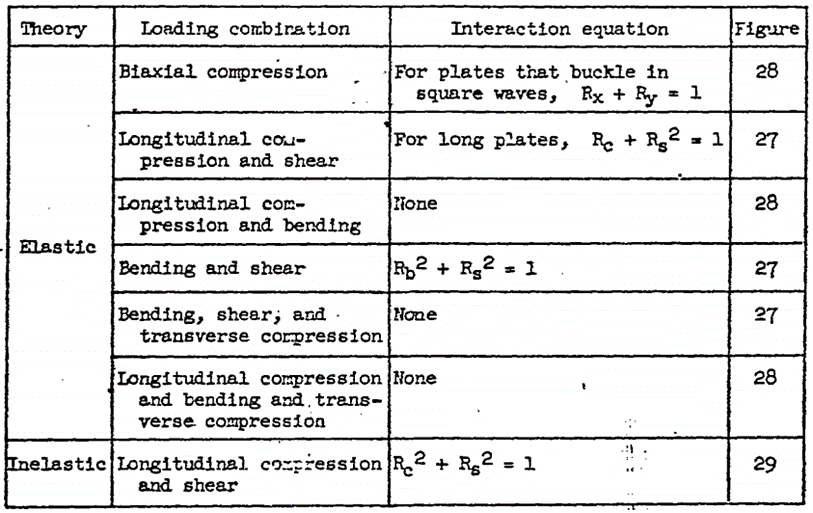Figure 15.2.10‑5: Rectangular Panel and Flange Buckling Interactions (NACA-TN-3781, 1957)

15.2.10.5. Triangular Panel buckling Coefficients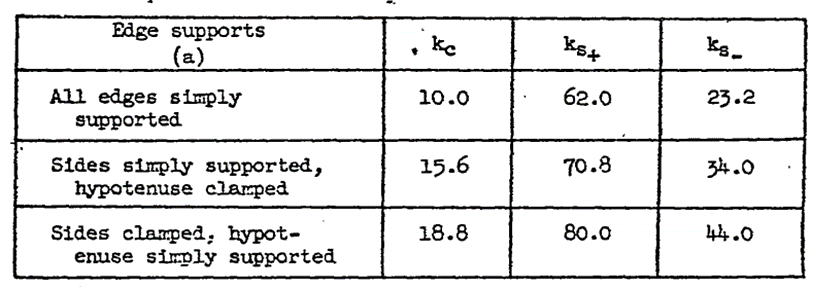Figure 15.2.10‑6: Rectangular Panel and Flange Buckling Interactions (NACA-TN-3781, 1957)

### 15.2.10. Panel and Flange Buckling Summary

Reference: Abbott, Richard. Analysis and Design of Composite and Metallic Flight Vehicle Structures 3 Edition, 2019.

15.2.10.1 Rectangular Panel Buckling Coefficients

(NACA-TN-3781, 1957) gives the following summary for minimum buckling coefficient figures (infinitely long Panels):Figure 15.2.10‑1: Rectangular Panel and Flange Buckling Stress Coefficient Summary  (NACA-TN-3781, 1957)

15.2.10.2. Rectangular Panel Plasticity Correction Factors

And the following summary for plasticity reduction factors:Figure 15.2.10‑2: Rectangular Panel and Flange Buckling Plasticity Correction Factors  (NACA-TN-3781, 1957)

15.2.10.3. Rectangular Panel Cladding Correction Factors

And the following summary for cladding reduction factors:Figure 15.2.10‑3: Rectangular Panel and Flange Buckling Cladding Correction Factors  (NACA-TN-3781, 1957)Figure 15.2.10‑4: Rectangular Panel and Flange Buckling Cladding Thicknesses  (NACA-TN-3781, 1957)

15.2.10.4. Rectangular Panel Buckling InteractionsFigure 15.2.10‑5: Rectangular Panel and Flange Buckling Interactions (NACA-TN-3781, 1957)

15.2.10.5. Triangular Panel buckling CoefficientsFigure 15.2.10‑6: Rectangular Panel and Flange Buckling Interactions (NACA-TN-3781, 1957)